#### Approximate solutions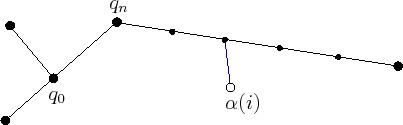Approximate solutions are much easier to construct, however, a resolution parameter is introduced. Each path segment can be approximated by inserting intermediate vertices along long segments, as shown in Figure 5.22. The intermediate vertices should be added each time a new sample,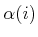, is inserted into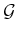. A parameter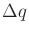can be defined, and intermediate samples are inserted to ensure that no two consecutive vertices inare ever further thanfrom each other. Using intermediate vertices, the interiors of the edges inare ignored when finding the nearest point in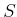. The approximate computation of NEAREST is performed by finding the closest vertex toin. This approach is by far the simplest to implement. It also fits precisely under the incremental sampling and searching framework from Section 5.4.1.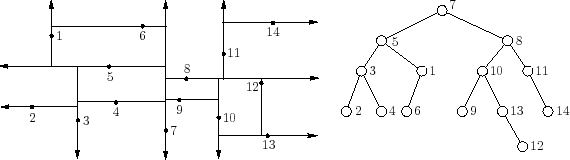When using intermediate vertices, the trade-offs are clear. The computation time for each evaluation of NEAREST is linear in the number of vertices. Increasing the number of vertices improves the quality of the approximation, but it also dramatically increases the running time. One way to recover some of this cost is to insert the vertices into an efficient data structure for nearest-neighbor searching. One of the most practical and widely used data structures is the Kd-tree [264,365,758]. A depiction is shown in Figure 5.23 for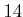points in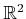. The Kd-tree can be considered as a multi-dimensional generalization of a binary search tree. The Kd-tree is constructed for points,, inas follows. Initially, sort the points with respect to thecoordinate. Take the median point,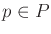, and divideinto two sets, depending on which side of a vertical line through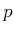the other points fall. For each of the two sides, sort the points by the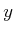coordinate and find their medians. Points are divided at this level based on whether they are above or below horizontal lines. At the next level of recursion, vertical lines are used again, followed by horizontal again, and so on. The same idea can be applied in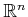by cycling through thecoordinates, instead of alternating betweenand, to form the divisions. In , the Kd-tree is extended to topological spaces that arise in motion planning and is shown to yield good performance for RRTs and sampling-based roadmaps. A Kd-tree ofpoints can be constructed in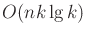time. Topological identifications must be carefully considered when traversing the tree. To find the nearest point in the tree to some given point, the query algorithm descends to a leaf vertex whose associated region contains the query point, finds all distances from the data points in this leaf to the query point, and picks the closest one. Next, it recursively visits those surrounding leaf vertices that are further from the query point than the closest point found so far [47,52]. The nearest point can be found in time logarithmic in.

Unfortunately, these bounds hide a constant that increases exponentially with the dimension,. In practice, the Kd-tree is useful in motion planning for problems of up to about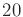dimensions. After this, the performance usually degrades too much. As an empirical rule, if there are more than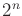points, then the Kd-tree should be more efficient than naive nearest neighbors. In general, the trade-offs must be carefully considered in a particular application to determine whether exact solutions, approximate solutions with naive nearest-neighbor computations, or approximate solutions with Kd-trees will be more efficient. There is also the issue of implementation complexity, which probably has caused most people to prefer the approximate solution with naive nearest-neighbor computations.

Steven M LaValle 2020-08-14